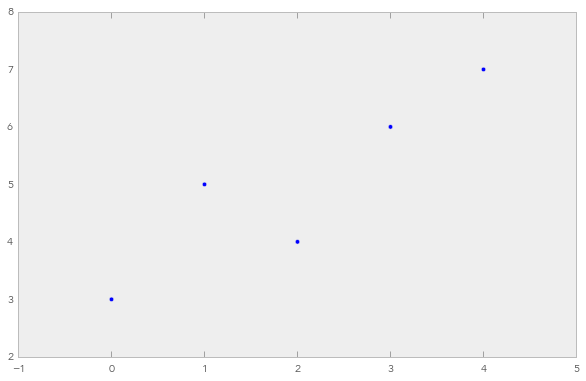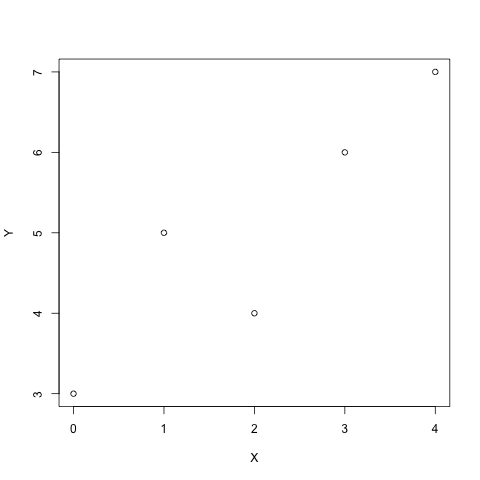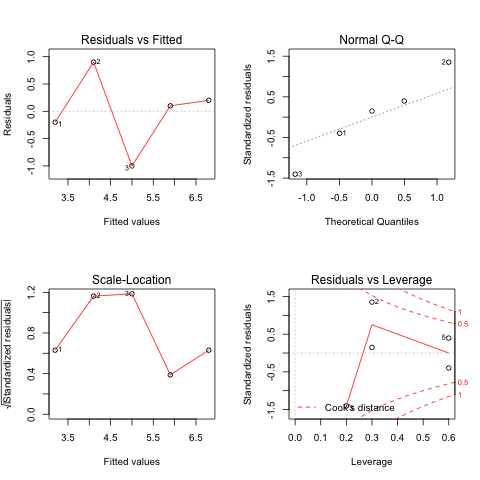# Using R Within the IPython Notebok¶

Using the rmagic extension, users can run R code from within the IPython Notebook. This example Notebook demonstrates this capability.

In :
%matplotlib inline


## Line magics¶

IPython has an rmagic extension that contains a some magic functions for working with R via rpy2. This extension can be loaded using the %load_ext magic as follows:

In :
%load_ext rmagic


A typical use case one imagines is having some numpy arrays, wanting to compute some statistics of interest on these arrays and return the result back to python. Let's suppose we just want to fit a simple linear model to a scatterplot.

In :
import numpy as np
import matplotlib.pyplot as plt
X = np.array([0,1,2,3,4])
Y = np.array([3,5,4,6,7])
plt.scatter(X, Y)

Out:
<matplotlib.collections.PathCollection at 0x107efe2d0>We can accomplish this by first pushing variables to R, fitting a model and returning the results. The line magic %Rpush copies its arguments to variables of the same name in rpy2. The %R line magic evaluates the string in rpy2 and returns the results. In this case, the coefficients of a linear model.

In :
%Rpush X Y
%R lm(Y~X)\$coef

Out:
array([ 3.2,  0.9])

We can check that this is correct fairly easily:

In :
Xr = X - X.mean(); Yr = Y - Y.mean()
slope = (Xr*Yr).sum() / (Xr**2).sum()
intercept = Y.mean() - X.mean() * slope
(intercept, slope)

Out:
(3.2000000000000002, 0.90000000000000002)

It is also possible to return more than one value with %R.

In :
%R resid(lm(Y~X)); coef(lm(X~Y))

Out:
array([-2.5,  0.9])

One can also easily capture the results of %R into python objects. Like R, the return value of this multiline expression (multiline in the sense that it is separated by ';') is the final value, which is the coef(lm(X~Y)). To pull other variables from R, there is one more magic.

There are two more line magics, %Rpull and %Rget. Both are useful after some R code has been executed and there are variables in the rpy2 namespace that one would like to retrieve. The main difference is that one returns the value (%Rget), while the other pulls it to self.shell.user_ns (%Rpull). Imagine we've stored the results of some calculation in the variable "a" in rpy2's namespace. By using the %R magic, we can obtain these results and store them in b. We can also pull them directly to user_ns with %Rpull. They are both views on the same data.

In :
b = %R a=resid(lm(Y~X))
%Rpull a
print(a)
assert id(b.data) == id(a.data)
%R -o a

[-0.2  0.9 -1.   0.1  0.2]


%Rpull is equivalent to calling %R with just -o

In :
%R d=resid(lm(Y~X)); e=coef(lm(Y~X))
%R -o d -o e
%Rpull e
print(d)
print(e)
import numpy as np
np.testing.assert_almost_equal(d, a)

[-0.2  0.9 -1.   0.1  0.2]
[ 3.2  0.9]


On the other hand %Rpush is equivalent to calling %R with just -i and no trailing code.

In :
A = np.arange(20)
%R -i A
%R mean(A)

Out:
array([ 9.5])

The magic %Rget retrieves one variable from R.

In :
%Rget A

Out:
array([ 0,  1,  2,  3,  4,  5,  6,  7,  8,  9, 10, 11, 12, 13, 14, 15, 16,
17, 18, 19], dtype=int32)

## Plotting and capturing output¶

R's console (i.e. its stdout() connection) is captured by ipython, as are any plots which are published as PNG files, as with %matplotlib inline. As a call to %R may produce a return value (see above) we must ask what happens to a magic like the one below. The R code specifies that something is published to the notebook. If anything is published to the notebook, that call to %R returns None.

In :
from __future__ import print_function
v1 = %R plot(X,Y); print(summary(lm(Y~X))); vv=mean(X)*mean(Y)
print('v1 is:', v1)
v2 = %R mean(X)*mean(Y)
print('v2 is:', v2)

Call:
lm(formula = Y ~ X)

Residuals:
1    2    3    4    5
-0.2  0.9 -1.0  0.1  0.2

Coefficients:
Estimate Std. Error t value Pr(>|t|)
(Intercept)   3.2000     0.6164   5.191   0.0139 *
X             0.9000     0.2517   3.576   0.0374 *
---
Signif. codes:  0 ‘***’ 0.001 ‘**’ 0.01 ‘*’ 0.05 ‘.’ 0.1 ‘ ’ 1

Residual standard error: 0.7958 on 3 degrees of freedom
Multiple R-squared:  0.81,	Adjusted R-squared: 0.7467
F-statistic: 12.79 on 1 and 3 DF,  p-value: 0.03739v1 is: [ 10.]
v2 is: [ 10.]


## What value is returned from %R?¶

Some calls have no particularly interesting return value, the magic %R will not return anything in this case. The return value in rpy2 is actually NULL so %R returns None.

In :
v = %R plot(X,Y)
assert v == NoneAlso, if the return value of a call to %R (in line mode) has just been printed to the console, then its value is also not returned.

In :
v = %R print(X)
assert v == None

 0 1 2 3 4


But, if the last value did not print anything to console, the value is returned:

In :
v = %R print(summary(X)); X
print('v:', v)

   Min. 1st Qu.  Median    Mean 3rd Qu.    Max.
0       1       2       2       3       4

v: [0 1 2 3 4]


The return value can be suppressed by a trailing ';' or an -n argument.

In :
%R -n X

In :
%R X;


## Cell level magic¶

Often, we will want to do more than a simple linear regression model. There may be several lines of R code that we want to use before returning to python. This is the cell-level magic.

For the cell level magic, inputs can be passed via the -i or --inputs argument in the line. These variables are copied from the shell namespace to R's namespace using rpy2.robjects.r.assign. It would be nice not to have to copy these into R: rnumpy ( http://bitbucket.org/njs/rnumpy/wiki/API ) has done some work to limit or at least make transparent the number of copies of an array. This seems like a natural thing to try to build on. Arrays can be output from R via the -o or --outputs argument in the line. All other arguments are sent to R's png function, which is the graphics device used to create the plots.

We can redo the above calculations in one ipython cell. We might also want to add some output such as a summary from R or perhaps the standard plotting diagnostics of the lm.

In :
%%R -i X,Y -o XYcoef
XYlm = lm(Y~X)
XYcoef = coef(XYlm)
print(summary(XYlm))
par(mfrow=c(2,2))
plot(XYlm)

Call:
lm(formula = Y ~ X)

Residuals:
1    2    3    4    5
-0.2  0.9 -1.0  0.1  0.2

Coefficients:
Estimate Std. Error t value Pr(>|t|)
(Intercept)   3.2000     0.6164   5.191   0.0139 *
X             0.9000     0.2517   3.576   0.0374 *
---
Signif. codes:  0 ‘***’ 0.001 ‘**’ 0.01 ‘*’ 0.05 ‘.’ 0.1 ‘ ’ 1

Residual standard error: 0.7958 on 3 degrees of freedom
Multiple R-squared:  0.81,	Adjusted R-squared: 0.7467
F-statistic: 12.79 on 1 and 3 DF,  p-value: 0.03739## Passing data back and forth¶

Currently, data is passed through RMagics.pyconverter when going from python to R and RMagics.Rconverter when going from R to python. These currently default to numpy.ndarray. Future work will involve writing better converters, most likely involving integration with http://pandas.sourceforge.net.

Passing ndarrays into R seems to require a copy, though once an object is returned to python, this object is NOT copied, and it is possible to change its values.

In :
seq1 = np.arange(10)

In :
%%R -i seq1 -o seq2
seq2 = rep(seq1, 2)
print(seq2)

  0 1 2 3 4 5 6 7 8 9 0 1 2 3 4 5 6 7 8 9

In :
seq2[::2] = 0
seq2

Out:
array([0, 1, 0, 3, 0, 5, 0, 7, 0, 9, 0, 1, 0, 3, 0, 5, 0, 7, 0, 9], dtype=int32)
In :
%%R
print(seq2)

  0 1 0 3 0 5 0 7 0 9 0 1 0 3 0 5 0 7 0 9


Once the array data has been passed to R, modifring its contents does not modify R's copy of the data.

In :
seq1 = 200
%R print(seq1)

  0 1 2 3 4 5 6 7 8 9


But, if we pass data as both input and output, then the value of "data" in user_ns will be overwritten and the new array will be a view of the data in R's copy.

In :
print(seq1)
%R -i seq1 -o seq1
print(seq1)
seq1 = 200
%R print(seq1)
seq1_view = %R seq1
assert(id(seq1_view.data) == id(seq1.data))

[200   1   2   3   4   5   6   7   8   9]
[200   1   2   3   4   5   6   7   8   9]

  200   1   2   3   4   5   6   7   8   9


## Exception handling¶

Exceptions are handled by passing back rpy2's exception and the line that triggered it.

In :
try:
%R -n nosuchvar
except Exception as e:
print(e)
pass

parsing and evaluating line "nosuchvar".
R error message: "Error in eval(expr, envir, enclos) : object 'nosuchvar' not found
"
R stdout:"Error in eval(expr, envir, enclos) : object 'nosuchvar' not found
"



## Structured arrays and data frames¶

In R, data frames play an important role as they allow array-like objects of mixed type with column names (and row names). In bumpy, the closest analogy is a structured array with named fields. In future work, it would be nice to use pandas to return full-fledged DataFrames from rpy2. In the mean time, structured arrays can be passed back and forth with the -d flag to %R, %Rpull, and %Rget

In :
datapy= np.array([(1, 2.9, 'a'), (2, 3.5, 'b'), (3, 2.1, 'c')],
dtype=[('x', '<i4'), ('y', '<f8'), ('z', '|S1')])

In :
%%R -i datapy -d datar
datar = datapy

In :
datar

Out:
array([(1, 2.9, 'a'), (2, 3.5, 'b'), (3, 2.1, 'c')],
dtype=[('x', '<i4'), ('y', '<f8'), ('z', '|S1')])
In :
%R datar2 = datapy
%Rpull -d datar2
datar2

Out:
array([(1, 2.9, 'a'), (2, 3.5, 'b'), (3, 2.1, 'c')],
dtype=[('x', '<i4'), ('y', '<f8'), ('z', '|S1')])
In :
%Rget -d datar2

Out:
array([(1, 2.9, 'a'), (2, 3.5, 'b'), (3, 2.1, 'c')],
dtype=[('x', '<i4'), ('y', '<f8'), ('z', '|S1')])

For arrays without names, the -d argument has no effect because the R object has no colnames or names.

In :
Z = np.arange(6)
%R -i Z
%Rget -d Z

Out:
array([0, 1, 2, 3, 4, 5], dtype=int32)

For mixed-type data frames in R, if the -d flag is not used, then an array of a single type is returned and its value is transposed. This would be nice to fix, but it seems something that should be fixed at the rpy2 level (See: https://bitbucket.org/lgautier/rpy2/issue/44/numpyrecarray-as-dataframe)

In :
%Rget datar2

Out:
array([['1', '2', '3'],
['2', '3', '2'],
['a', 'b', 'c']],
dtype='|S1')